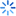• 签到
•苹果/安卓/wp

•苹果/安卓/wp

客户端
normcdf的逆求解（matlab）
• 统计与数据分析
• 经济学
• 管理学
• 金融学
• 财会类
• 国际贸易类
• 考研考博与考证
• 找数据和资料
• 求职与职场
• 学术与投稿类
• 社会生活
• 其它

normcdf的逆求解（matlab）1438413
matlab中函数normcdf（a,0,1），由a可以知道正态分布的累积概率，比如当a=0的时候，正态分布的累积概率为0.5.现在我是想反过来求解，就是知道了正态分布的累积概率为0.5的时候，怎么求a=0.。。。。急急急syslon

norminv函数，具体用法楼主可以参考help文件

[size=1.2em]Normal inverse cumulative distribution function

[url=]collapse all in page[/url]

Syntax

X = norminv(P,mu,sigma)
[X,XLO,XUP] = norminv(P,mu,sigma,pcov,alpha)

Description

X = norminv(P,mu,sigma) computes the inverse of the normal cdf using the corresponding mean mu and standard deviation sigma at the corresponding probabilities in P. P, mu, and sigma can be vectors, matrices, or multidimensional arrays that all have the same size. A scalar input is expanded to a constant array with the same dimensions as the other inputs. The parameters in sigma must be positive, and the values in P must lie in the interval [0 1].

[X,XLO,XUP] = norminv(P,mu,sigma,pcov,alpha) produces confidence bounds for X when the input parameters mu and sigma are estimates.pcov is the covariance matrix of the estimated parameters. alpha specifies 100(1 - alpha)% confidence bounds. The default value of alpha is 0.05.XLO and XUP are arrays of the same size as X containing the lower and upper confidence bounds.加载中...